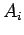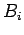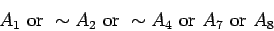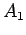Next: Converting (SP) to (SSP) Up: Subset-Sum Problems and NP-Completeness Previous: Subset-Sum Problems and NP-Completeness   Contents

## The Satisfiability Problem

We will use capitalletters,, to stand for logical variables. These stand for statements like 221 is a prime number'' or TH is the most common two-letter sequence in English,'' which are either true or false, i. e., these variables have values of either T or F.(not'') is the statement thatis false, so it has value T ifhas value F, andhas value F ifhas value T. We will also be interested in more elaborate formulas:This formula says that eitheris true oris false, oris false, etc. The value of this formula will be T unless,,,,. Thus, there is only one way in which the formula will be false.

Figure 1 illustrates a satisfiability problem.We want to assign T, F to all the variables so that all of the formulas have value T. Even in this small example, it may take you a minute or so to find such an assignment.Next: Converting (SP) to (SSP) Up: Subset-Sum Problems and NP-Completeness Previous: Subset-Sum Problems and NP-Completeness   Contents
Translated from LaTeX by Scott Sutherland
2002-12-14